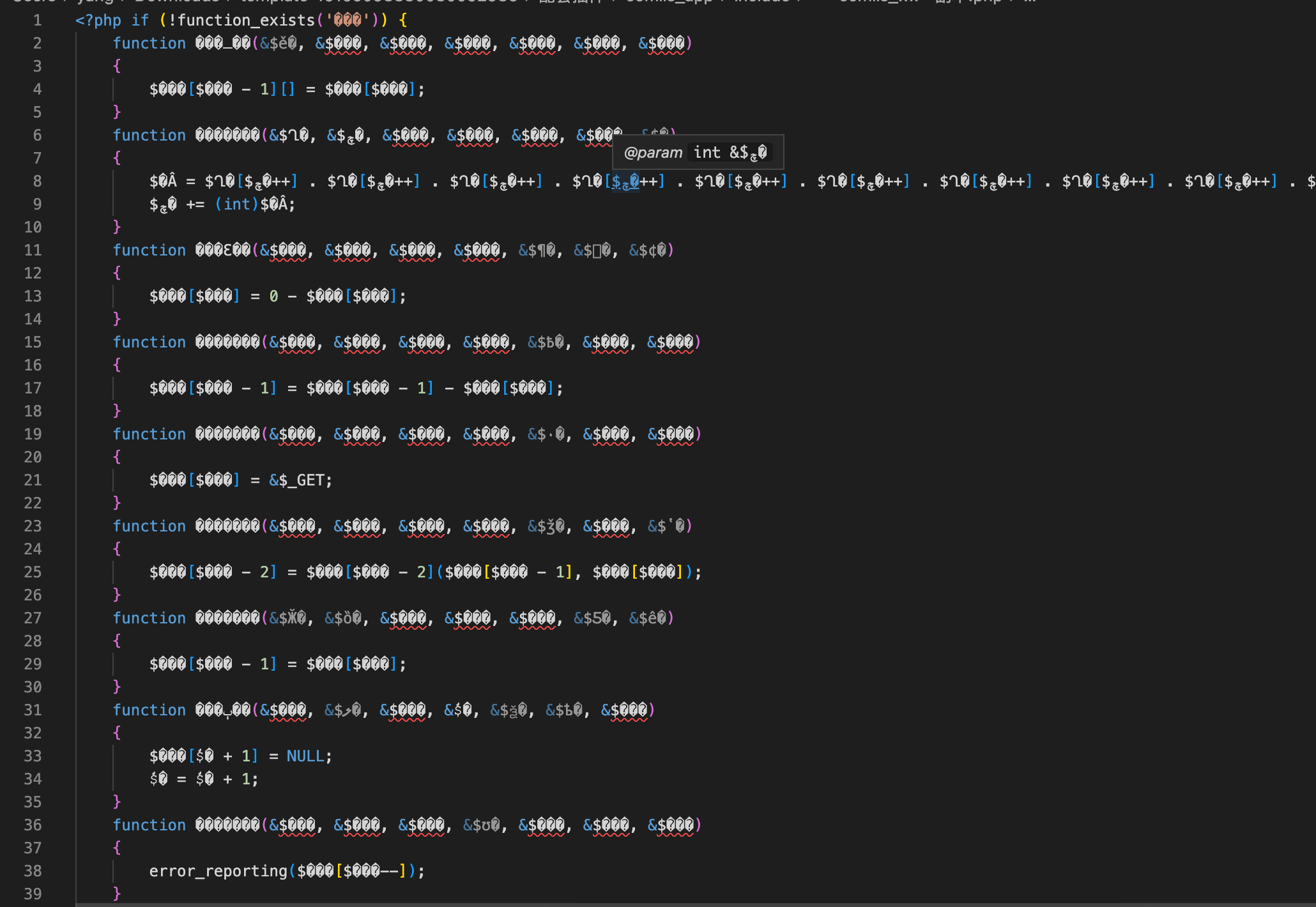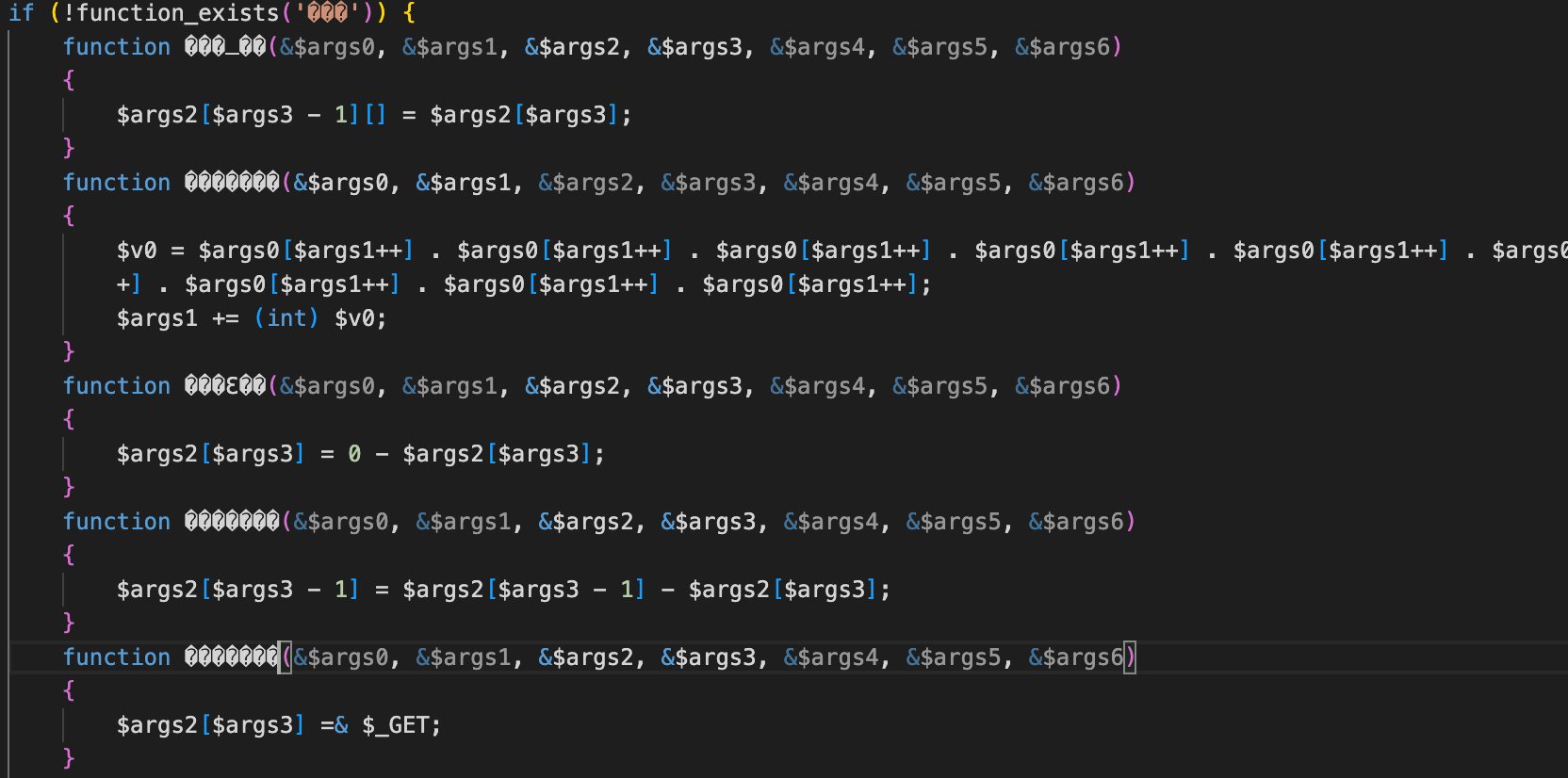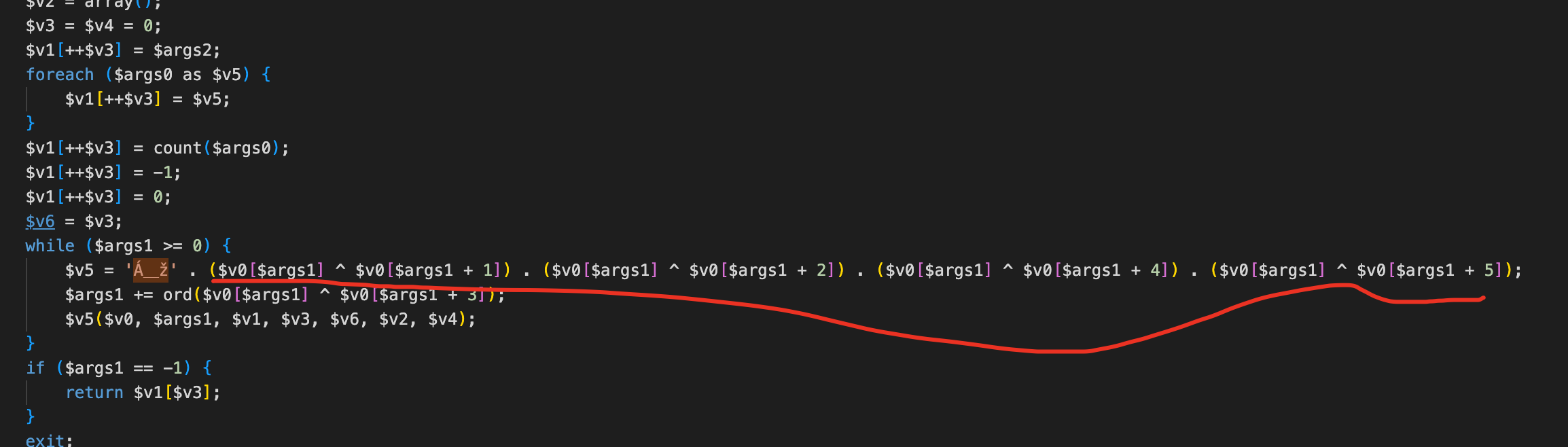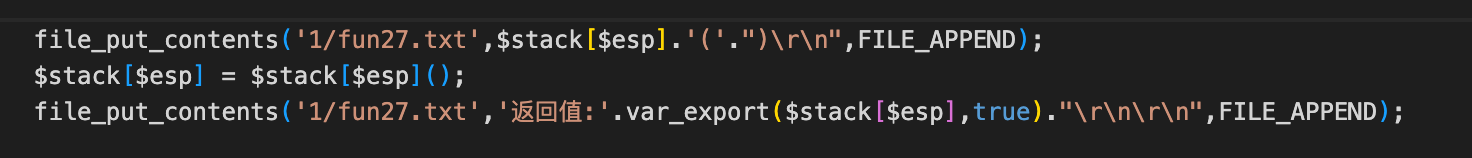``````<?php

use PhpParser\Error;
use PhpParser\ParserFactory;
use PhpParser\PrettyPrinter;

ini_set('xdebug.max_nesting_level', 10000);

\$input_file = \$argv;
\$output_file = \$argv . '.formatted.php';

\$code = file_get_contents(\$input_file);

// 解析代码
\$parser = (new ParserFactory)->create(ParserFactory::PREFER_PHP7);
try {
\$ast = \$parser->parse(\$code);
} catch (Error \$error) {
echo "Parse error: {\$error->getMessage()}\n";
return;
}

// 格式化代码
\$prettyPrinter = new PrettyPrinter\Standard;
\$prettyCode = \$prettyPrinter->prettyPrintFile(\$ast);

file_put_contents(\$output_file, \$prettyCode);````````function func1(&\$arg0, &\$eip, &\$arg2, &\$arg3, &\$arg4, &\$arg5, &\$arg6);``

``function func0(\$arg0, \$eip, \$arg2 = null)``

``func0(array(), 0)``

``````function func1(\$memory, \$eip, \$stack, \$esp, \$ebp, \$error_level_stack, \$error_level_stack_pointer) {
// 这里都没有 return 都是通过引用参数返回的
}

function func_call(array \$args, \$eip, \$ret = null)
{
static \$memory;
if (strlen(\$memory) == 0) {
\$memory = '......';
}
\$stack = array();
\$error_level_stack = array(); // 用于保存 error_reporting level
\$esp = \$error_level_stack_pointer = 0;
\$stack[++\$esp] = \$ret;
foreach (\$args as \$item) {
\$stack[++\$esp] = \$item;
}
\$stack[++\$esp] = count(\$args);
\$stack[++\$esp] = -1;
\$stack[++\$esp] = 0;
\$ebp = \$esp;
while (\$eip >= 0) {
\$func = 'func' . (\$memory[\$eip] ^  \$memory[\$eip + 1]) . (\$memory[\$eip] ^  \$memory[\$eip + 4]) . (\$memory[\$eip] ^  \$memory[\$eip + 5]);
\$eip += ord(\$memory[\$eip] ^ \$memory[\$eip + 3]);
\$func(\$memory, \$eip, \$stack, \$esp, \$ebp, \$error_level_stack, \$error_level_stack_pointer);
}
if (\$eip == -1) {
return \$stack[\$esp];
}
}

func_call(array(), 0);``````

``````use PhpParser\Node;
use PhpParser\NodeTraverser;
use PhpParser\NodeVisitorAbstract;

// 变量名替换
class VariableRenameNodeVisitor extends NodeVisitorAbstract
{
protected \$paramsMap = [];

public function __construct(&\$params_map)
{
\$this->paramsMap = \$params_map;
}

public function generateNewVariableName()
{
\$values = array_values(\$this->paramsMap);
\$i = 0;
while (in_array('v' . \$i, \$values)) {
++\$i;
}
return 'v' . \$i;
}

public function leaveNode(Node \$node)
{
if (\$node instanceof Node\Expr\Variable) {
if (!isset(\$this->paramsMap[\$node->name])) {
\$this->paramsMap[\$node->name] = \$this->generateNewVariableName();
}
\$node->name = \$this->paramsMap[\$node->name];
}
}
}

class FunctionParamsRenameNodeVisitor extends NodeVisitorAbstract
{
public function leaveNode(Node \$node)
{
if (\$node instanceof Node\Stmt\Function_) {
\$params_map = [];
foreach (\$node->params as \$i => &\$param) {
\$params_map[\$param->name] = 'arg' . \$i;
\$param->name = \$params_map[\$param->name];
}
\$visitor = new VariableRenameNodeVisitor(\$params_map);
\$traverser = new NodeTraverser;
\$node->stmts = \$traverser->traverse(\$node->stmts);
}
}
}

\$traverser = new NodeTraverser;
\$ast = \$traverser->traverse(\$ast);```````\$memory` 赋值语句之后插入 `file_put_contents`

``\$memory = '......';file_put_contents('opcode.php', \$memory);``

``\$memory = file_get_contents('opcode.php');``

``````function func51(&\$memory, &\$eip, &\$stack, &\$esp, &\$ebp, &\$error_level_stack, &\$error_level_stack_pointer)
{
eval("function " . \$stack[\$esp] . '(){return func65(func_get_args(),' . (int) (\$memory[\$eip++] . \$memory[\$eip++] . \$memory[\$eip++] . \$memory[\$eip++] . \$memory[\$eip++] . \$memory[\$eip++] . \$memory[\$eip++] . \$memory[\$eip++] . \$memory[\$eip++] . \$memory[\$eip++] . \$memory[\$eip++] . \$memory[\$eip++]) . ');}');
}
function func65(array \$args, \$eip, \$ret = null)
{
static \$memory, \$func_name_map;
if (strlen(\$memory) == 0) {
\$memory = file_get_contents('1.php.opcode.bin');
\$func_name_map = include '2.php.formatted.php.func_name_map.php';
}
\$stack = [];
\$error_level_stack = [];
\$esp = \$error_level_stack_pointer = 0;
\$stack[++\$esp] = \$ret;
foreach (\$args as \$item) {
\$stack[++\$esp] = \$item;
}
\$stack[++\$esp] = count(\$args);
\$stack[++\$esp] = -1;
\$stack[++\$esp] = 0;
\$ebp = \$esp;
while (\$eip >= 0) {
\$func = base64_decode('zb+8') . (\$memory[\$eip] ^ \$memory[\$eip + 1]) . (\$memory[\$eip] ^ \$memory[\$eip + 2]) . (\$memory[\$eip] ^ \$memory[\$eip + 4]) . (\$memory[\$eip] ^ \$memory[\$eip + 5]);
\$eip += ord(\$memory[\$eip] ^ \$memory[\$eip + 3]);
if (isset(\$func_name_map[\$func])) {
\$func = \$func_name_map[\$func];
}
\$func(\$memory, \$eip, \$stack, \$esp, \$ebp, \$error_level_stack, \$error_level_stack_pointer);
}
if (\$eip == -1) {
return \$stack[\$esp];
}
exit;
}
include 'func_map.php';``````

`opcode.php`是关键的代码，`func_map`是函数名映射地图代码！`` echo 'hellow word';``